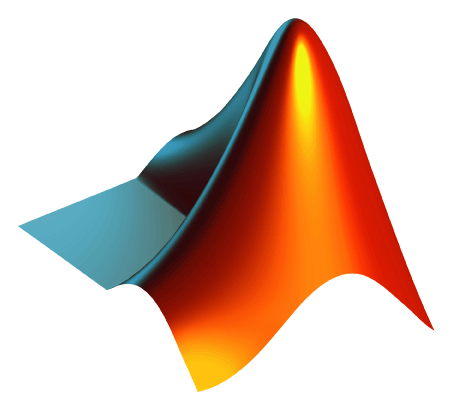Community Profile# Jos Martin

### MathWorks

Last seen: 5 days ago Active since 2011

Director Of Engineering - Cloud Integration and Parallel Computing.#### Statistics

All
•••••••#### Content Feed

View by

Channel

BBH Internal Network

4 months ago

Channel

router-01-external-ping

9 months ago

Channel

router-01

10 months ago

Solved

Finding Perfect Squares
Given a vector of numbers, return true if one of the numbers is a square of one of the other numbers. Otherwise return false. E...

2 years ago

Solved

Piecewise linear interpolation
Given an Mx2 vector and a row of M-1 integers, output a two column vector that linearly interpolates Y times between each succes...

3 years ago

Solved

How to subtract?
*&plusmn &plusmn &plusmn &plusmn &plusmn &plusmn &plusmn &plusmn &plusmn &plusmn &plusmn* * Imagine you need to subtract one...

4 years ago

Submitted

Port Passing Problem
Monte-carlo and analytic investigation of a random walk processSubmitted

Hydrogenic Wavefunction Visulization Tool
Visualize hydrogenic wave-functions in 3D and watch animations of atomic state changesSubmitted

Cluster setup scripts
Shell scripts to easily control a MATLAB distributing computing cluster

Submitted

Visual Timing Report for Distributed Tasks
Create a visual representation of when and where each task in a distributed computing job executes.Solved

Is my wife right?
Regardless of input, output the string 'yes'.

5 years ago

Channel

Burwell Router

5 years ago

Channel

CurlTest

6 years ago

Channel

6 years ago

Solved

Select every other element of a vector
Write a function which returns every other element of the vector passed in. That is, it returns the all odd-numbered elements, s...

9 years ago

GPU computing with OS X 10.8 and retina macbook
We believe that the hang you are seeing relates to the CUDA driver recompiling all of the GPU libraries to run on the particular...

9 years ago | 4

Matlab not recognizing cuda driver 5
There appears to be an interaction between "automatic graphics switching" in Mountain Lion and the underlying NVIDIA drivers. Co...

9 years ago | 0

Solved

Function composition - harder
Write a function that accepts an arbitrary number of function handles f_1, f_2, ..., f_n and returns the composition h. That is,...

9 years ago

Solved

Given a and b, return the sum a+b in c.

9 years ago

Solved

Determine if input is odd
Given the input n, return true if n is odd or false if n is even.

9 years ago

Solved

Make a checkerboard matrix
Given an integer n, make an n-by-n matrix made up of alternating ones and zeros as shown below. The a(1,1) should be 1. Example...

9 years ago

Solved

Find the sum of all the numbers of the input vector
Find the sum of all the numbers of the input vector x. Examples: Input x = [1 2 3 5] Output y is 11 Input x ...

9 years ago

Solved

Make the vector [1 2 3 4 5 6 7 8 9 10]
In MATLAB, you create a vector by enclosing the elements in square brackets like so: x = [1 2 3 4] Commas are optional, s...

9 years ago

Solved

Times 2 - START HERE
Try out this test problem first. Given the variable x as your input, multiply it by two and put the result in y. Examples:...

9 years ago